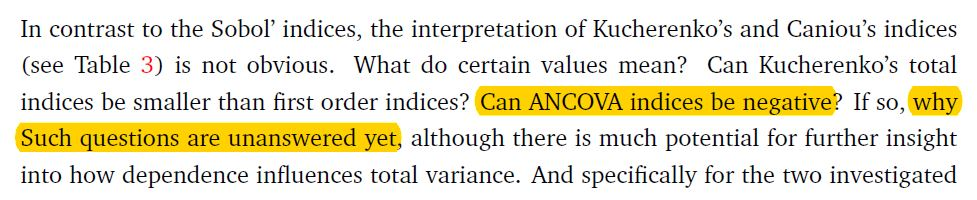# ANCOVA indices-How to have robust sensitivity analysis?

Dear UQLab

First of all, thank you very much for the implementation of the ANCOVA indices to establish sensitivity analysis with dependent marginals.
Second, I have two problems:

1. What’s the meaning of NEGATIVE first order ANCOVA indices?
2. Is it “wrong” to have big (~10^14) total variance in the sensitivity analysis using the first order ANCOVA indices?

Finally, Thanks for your enjoyable UQLab.
Best regards
Ali

Dear @ali

You can find a detailed analysis and comparisons for sensitivity indices in case of dependent inputs in the master thesis by P. Wiederkehr (2018) and a nice literature review. This is still a cumbersome problem, for which certain indices, although mathematically well-defined, cannot really be interpreted. This master’s thesis gives some kind of recommendations.

To understand the negative indices, just think about the variance of a sum of correlated variables (linear case), where the latter have negative covariances: the total variance may be smaller than the sum of the variances of each individual variable. The cross-terms are negative, which would translate, after normalization, into negative indices. Of course it is a bit more tricky, but this simple example gives at least an intuition.

Regarding high variance of the output, it depends on your problem and the units of your quantity of interest. In mechanical/civil engineering, if you compute stresses in Pascal (Pa), they can easily get values of 3\cdot 10^8, i.e 300 MPa. With 10% coefficient of variance, this would lead to a standard deviation of 3\cdot 10^7 Pa and a variance of 9\cdot 10^{14} Pa \!{}^2. So it is totally possible.

Best regards
Bruno

1 Like

Dear Prof. @bsudret

I read the mentioned thesis before asking this question. It studied good examples.
Unfortunately, I have the problem of interpretation of my sensitivity analysis. I really appreciate your recommendations.
For instance, I get the value of -9.819E-06 in one of my random variables (RV) by the first order ANCOVA sensitivity analysis. Can I interpret and substitute this value with ZERO or the absolute value?!
Besides, based on the mentioned study in p. 15, there is still some lack of knowledge in the context of ANCOVA indices!.In a sentence, what are your recommendations for us to do sensitivity analysis with dependent RVs?

I am most thankful for the sustainable supports of UQTeam.
Best regards
Ali

Dear UQLab